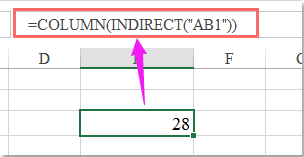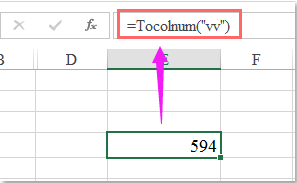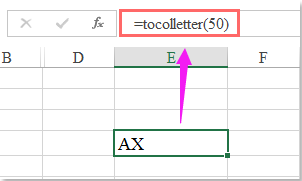## How to convert column letter to number or vice versa in Excel?

This article, I will talk about how to convert column letter to column number or convert column number to letter. Such as, convert column letter AA to the number 27, or convert column number 100 to letter CV. To get the solution with following methods.

Convert column letter to number or vice versa with formulas

Convert column letter to number or vice versa with User Defined Function

####Convert column letter to number or vice versa with formulas

To convert the column letter or label to number or vice versa, the below formulas may help you, please do as this:

Convert column letter to column number:

Please enter this formula: =COLUMN(INDIRECT("AB1")) into a blank cell where you want to locate the converted result, and then press Enter key to get the column number:Note: In the above formula, AB is the column letter that you want to get the column number based on, you can change it to your need.

Convert column number to column letter:

Please type this formula: =SUBSTITUTE(ADDRESS(1,200,4),1,"") into a blank cell to get the result, and then press Enter to get the column letter as follows:Note: In the above formula, the red number 200 is the column number that you want to convert to column letter, you can change it to your need.

####Convert column letter to number or vice versa with User Defined Function

Except the formulas, here, I can also introduce the User Defined Function to deal with this task.

Convert column letter to column number:

1. Hold down the ALT + F11 keys, then it opens the Microsoft Visual Basic for Applications window.

2. Click Insert > Module, and paste the following code in the Module Window.

VBA code: convert column letter to column number:

``````Public Function ToColNum(ColN)
ToColNum = Range(ColN & 1).Column
End Function
``````

3. Then save and close this code, go back to the worksheet, and enter this formula: =Tocolnum("VV") (VV is the column letter that you want to convert number. ) into a blank cell, and press Enter key to get the column number, see screenshot:Convert column number to column letter:

1. Hold down the ALT + F11 keys, then it opens the Microsoft Visual Basic for Applications window.

2. Click Insert > Module, and paste the following code in the Module Window.

VBA code: convert column number to column letter:

``````Public Function ToColletter(Collet)
End Function
``````

3. Then save and close this code, go back to the worksheet, and enter this formula: =ToColletter(50) (50 is the column number that you want to convert to letter. ) into a blank cell, and press Enter key to get the column number, see screenshot:### Best Office Productivity Tools

 Popular Features: Find, Highlight or Identify Duplicates   |  Delete Blank Rows   |  Combine Columns or Cells without Losing Data   |   Round without Formula ... Super Lookup: Multiple Criteria VLookup  |   Multiple Value VLookup  |   VLookup Across Multiple Sheets   |   Fuzzy Lookup .... Advanced Drop-down List: Quickly Create Drop Down List   |  Dependent Drop Down List   |  Multi-select Drop Down List .... Column Manager: Add a Specific Number of Columns  |  Move Columns  |  Toggle Visibility Status of Hidden Columns  |  Compare Ranges & Columns ... Featured Features: Grid Focus   |  Design View   |   Big Formula Bar   |  Workbook & Sheet Manager   |  Resource Library (Auto Text)   |  Date Picker   |  Combine Worksheets   |  Encrypt/Decrypt Cells   |  Send Emails by List   |  Super Filter   |   Special Filter (filter bold/italic/strikethrough...) ... Top 15 Toolsets:  12 Text Tools (Add Text, Remove Characters, ...)   |   50+ Chart Types (Gantt Chart, ...)   |   40+ Practical Formulas (Calculate age based on birthday, ...)   |   19 Insertion Tools (Insert QR Code, Insert Picture from Path, ...)   |   12 Conversion Tools (Numbers to Words, Currency Conversion, ...)   |   7 Merge & Split Tools (Advanced Combine Rows, Split Cells, ...)   |   ... and more

Supercharge Your Excel Skills with Kutools for Excel, and Experience Efficiency Like Never Before. Kutools for Excel Offers Over 300 Advanced Features to Boost Productivity and Save Time.  Click Here to Get The Feature You Need The Most...#### Office Tab Brings Tabbed interface to Office, and Make Your Work Much Easier

• Enable tabbed editing and reading in Word, Excel, PowerPoint, Publisher, Access, Visio and Project.
• Open and create multiple documents in new tabs of the same window, rather than in new windows.
• Increases your productivity by 50%, and reduces hundreds of mouse clicks for you every day!Rated 5 out of 5 · 1 ratings
This comment was minimized by the moderator on the site
Public Function AlphabetInteger(ByVal letter As String) As Integer
Dim N As Integer
letter = Strings.UCase(letter)
If letter = "A" Then
N = 1
ElseIf letter = "B" Then
N = 2
ElseIf letter = "C" Then
N = 3
ElseIf letter = "D" Then
N = 4
ElseIf letter = "E" Then
N = 5
ElseIf letter = "F" Then
N = 6
ElseIf letter = "G" Then
N = 7
ElseIf letter = "H" Then
N = 8
ElseIf letter = "I" Then
N = 9
ElseIf letter = "J" Then
N = 10
ElseIf letter = "K" Then
N = 11
ElseIf letter = "L" Then
N = 12
ElseIf letter = "M" Then
N = 13
ElseIf letter = "N" Then
N = 14
ElseIf letter = "O" Then
N = 15
ElseIf letter = "P" Then
N = 16
ElseIf letter = "Q" Then
N = 17
ElseIf letter = "R" Then
N = 18
ElseIf letter = "S" Then
N = 19
ElseIf letter = "T" Then
N = 20
ElseIf letter = "U" Then
N = 21
ElseIf letter = "V" Then
N = 22
ElseIf letter = "W" Then
N = 23
ElseIf letter = "X" Then
N = 24
ElseIf letter = "Y" Then
N = 25
ElseIf letter = "Z" Then
N = 26
Else
N = 0
End If
AlphabetInteger = N
End Function

Public Function ColumnNumber(ByVal columnLetter As String) As Integer
Dim I As Integer
Dim sLength As Integer
Dim N As Integer
Dim A As Integer
Dim P As Integer
Dim C As String

sLength = Len(columnLetter)
N = 0
For I = 1 To sLength
P = sLength - I
C = Strings.Mid(columnLetter, I, 1)
A = AlphabetInteger(C)
N = N + A * 26 ^ P
Next I
ColumnNumber = N
End Function
This comment was minimized by the moderator on the site
Thanks a lot.
Rated 5 out of 5
This comment was minimized by the moderator on the site
A to ZZ
=if(row()>26,char(row()/26+64)&char(mod(row(),26)+64),char(row()+64))
This comment was minimized by the moderator on the site
This formula does not work at rows that are multiples of 26. It gives B@ instead of AZ, C@ instead of BZ, D@ instead of CZ, etc.
Correction:= IF(ROW()>26,(IF(MOD(ROW(),26)=0,CHAR((ROW()-1)/26+64)&CHAR(MOD(ROW()-1,26)+65), CHAR(ROW()/26+64)&CHAR(MOD(ROW(),26)+64))),CHAR(ROW()+64))
This comment was minimized by the moderator on the site
thank you so much!!!...your formula to create the column letter to number really helps me a lot.
This comment was minimized by the moderator on the site
Function ToColletter(Collet) works as volatile function. If something changes anywhere in the sheet. It recalculate everywhere. In my case it makes 15 mil calculations and slowdown the calculation. Do you know how to fix it, if I dont want set application.calculation to manual and than back?
This comment was minimized by the moderator on the site
hola, cuando pego la formula = SUSTITUIR (DIRECCIÓN (1,200,4), 1, "") en una celda no hace nada, solo devuelve la formula, ya sea con 200 o cualquier otro numero
This comment was minimized by the moderator on the site
=CHAR(COLUMN( )+64)
This comment was minimized by the moderator on the site
Not better as it only works with 26 columns.
This comment was minimized by the moderator on the site
For so long I've used R1C1 cell referencing because I didn't know about the =SUBSTITUTE(ADDRESS(1,number,4),1,"") solution. Thank you!!
There are no comments posted here yet